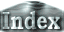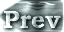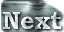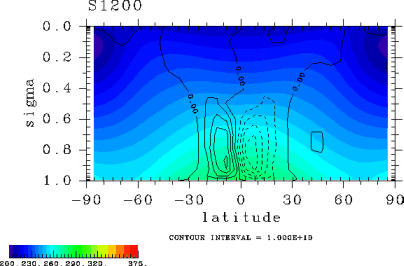# 3. Results### c. Changes in the Equilibrium State (Circulation Pattern)

A summary of the changes in atmospheric structure with increasing solar constant will be given below. We will first present cases in which the equilibrium states haves been reached.

Figure 1 provides an overview of the thermal and circulation structures of the atmosphere. This figure shows the meridional temperature distributions and mass stream functions for cases in which equilibrium states have been reached. The following observations may be made based on the figure.Figure 1: The meridional temperature distributions (K) and mass stream functions (kg/sec) in the cases of experiments S1200, S1380, S1500, S1550, and S1570.

Figures referred in this section are included in Appendix E and listed as follows:

 3.c. Changes in the Equilibrium State (Circulation Pattern)